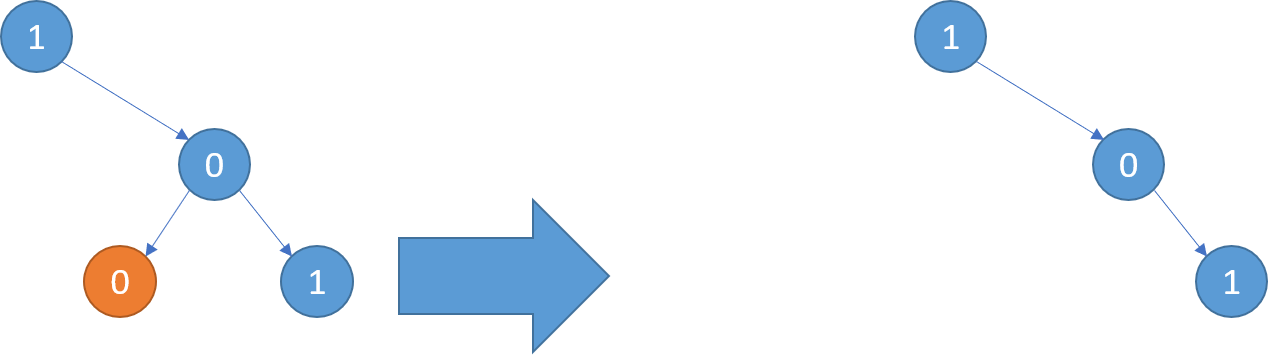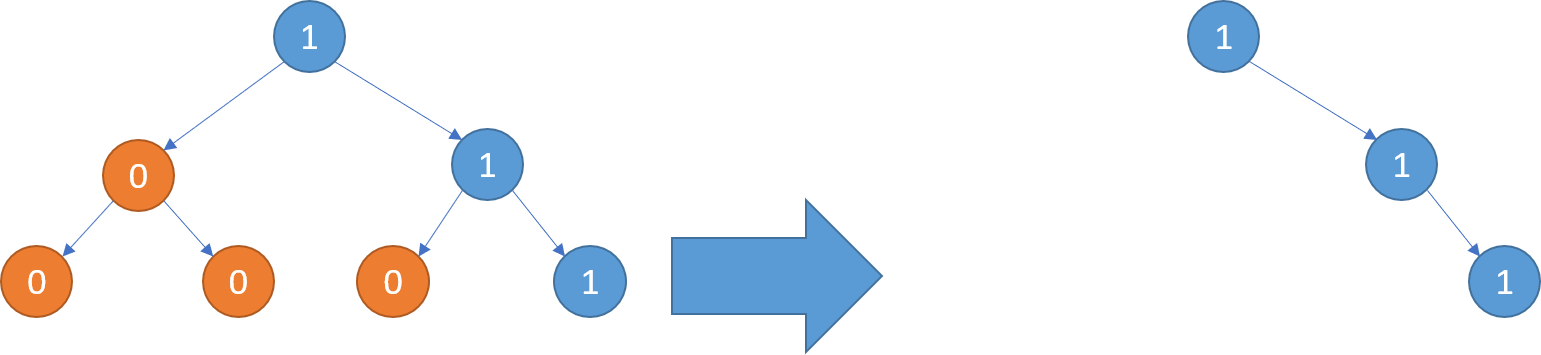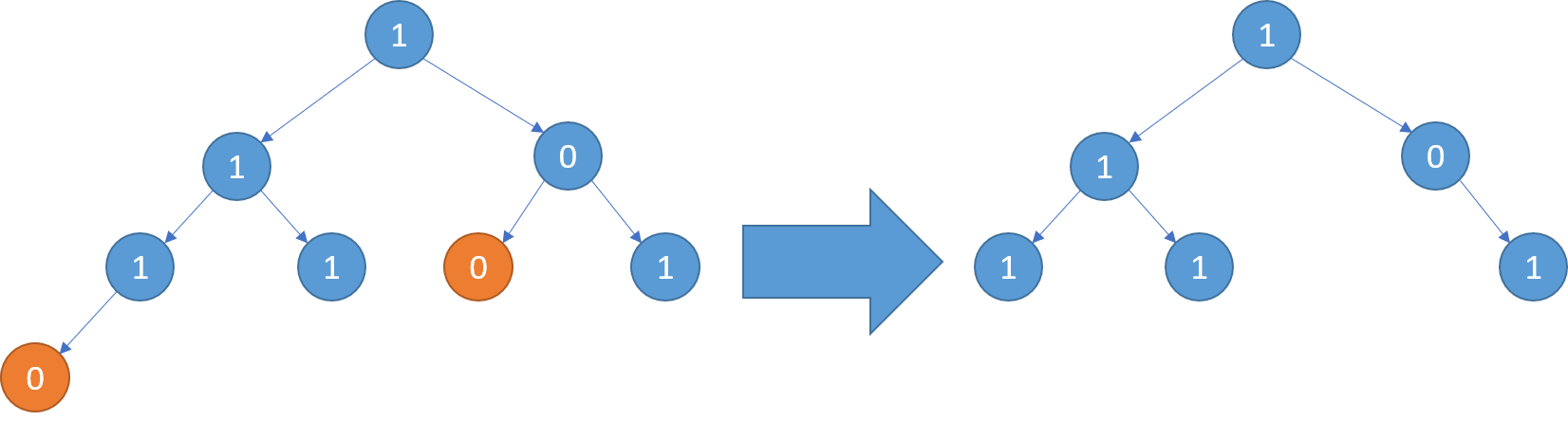We are given the head node `root` of a binary tree, where additionally every node’s value is either a 0 or a 1.

Return the same tree where every subtree (of the given tree) not containing a 1 has been removed.

(Recall that the subtree of a node X is X, plus every node that is a descendant of X.)

```Example 1:
Input: [1,null,0,0,1]
Output: [1,null,0,null,1]

Explanation:
Only the red nodes satisfy the property "every subtree not containing a 1".
The diagram on the right represents the answer.```
```Example 2:
Input: [1,0,1,0,0,0,1]
Output: [1,null,1,null,1]```
```Example 3:
Input: [1,1,0,1,1,0,1,0]
Output: [1,1,0,1,1,null,1]```

Note:

• The binary tree will have at most `200 nodes`.
• The value of each node will only be `0` or `1`.

Solution: (Recursion)

``````/**
* Definition for a binary tree node.
* function TreeNode(val, left, right) {
*     this.val = (val===undefined ? 0 : val)
*     this.left = (left===undefined ? null : left)
*     this.right = (right===undefined ? null : right)
* }
*/
/**
* @param {TreeNode} root
* @return {TreeNode}
*/
var pruneTree = function(root) {
// basic case:
if (root === null) return root;
root.left = pruneTree(root.left);
root.right = pruneTree(root.right);
// found 1 or has children then no need to remove
if (root.val === 1 || root.left || root.right)
return root;
else // remove node
return null;
};``````